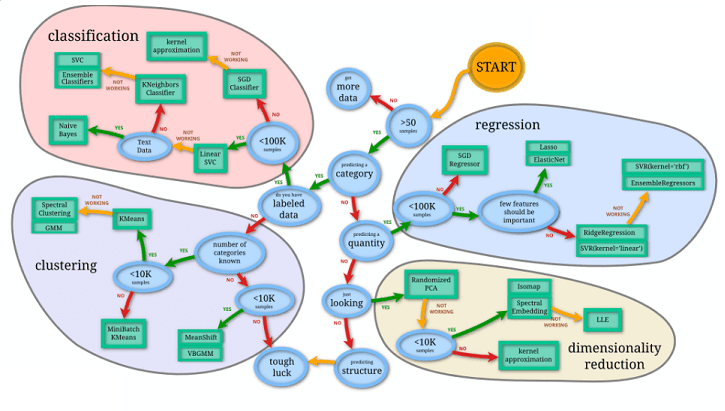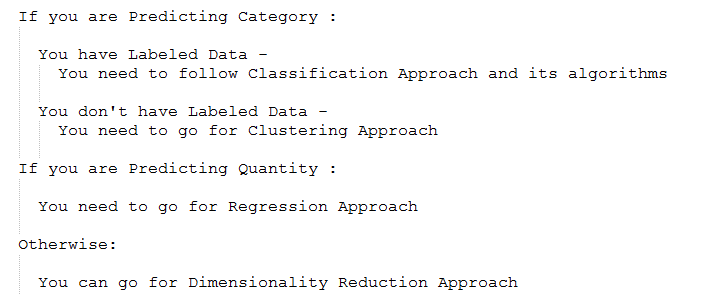Data Science

# Machine Learning Interview Questions

Machine Learning is a field focused on the automated identification of significant patterns within data. Its value lies in its ability to continuously learn from data and make future predictions. The effectiveness of Machine Learning is influenced by the quality and quantity of the data used for training. As a rapidly advancing discipline, Machine Learning offers promising career opportunities for skilled professionals. This comprehensive guide is specifically tailored to help you thoroughly prepare for your Machine Learning interviews, ensuring you are well-equipped to excel in the interview process.

Ready to dive in? Then let's get started!

## What is Machine learning?

Machine learning is a scientific discipline that employs probability theory to create models based on sample data, enabling the model to make predictions or devise strategies for new data. It involves the study of algorithms and statistical models that allow computers to learn from and make decisions or predictions without being explicitly programmed for each task. By using sample data, machine learning algorithms can generalize and adapt to new inputs, enhancing their predictive capabilities and strategic insights.

## Why do you need machine learning?

Machine learning is essential for tackling tasks that are too challenging for humans to develop directly. These tasks often involve processing massive datasets or performing complex calculations that would be impractical for humans to handle manually, considering all the intricate nuances. Machine learning provides a solution by using experience and continuously improving performance through iterative learning processes. By employing performance metrics, machine learning algorithms can refine their capabilities and adapt to new data, making them highly valuable for solving intricate and data-intensive problems.

## Difference between Artificial Intelligence and Machine Learning?

Artificial Intelligence (AI) is a comprehensive field aimed at emulating human behavior and intelligence. It encompasses various methods and approaches that can achieve this goal. Any technique capable of replicating human-like intelligence falls under the domain of Artificial Intelligence. Machine Learning, on the other hand, is a specific subset of AI that operationalizes AI by extracting patterns from data and using these patterns to make predictions or decisions. It enables machines to learn from data without explicit programming, allowing them to adapt and improve their performance over time. In essence, Machine Learning is a powerful tool within the broader scope of Artificial Intelligence, enabling systems to learn from experience and data to perform human-like tasks.

## What are Different Types of Machine Learning algorithms?

There some variations of how to define the types of ML Algorithms but commonly they can be categories according to their purpose and following are the main categories:

1. Supervised Learning
2. Unsupervised Learning
3. Semi-supervised Learning
4. Reinforcement Learning

Based on the above categories, here is the list of commonly used machine learning algorithms.

1. Linear Regression
2. Logistic Regression
3. Decision Tree
4. Support Vector Machines (SVM)
5. Naive Bayes
6. kNN
7. K-Means
8. Random Forest
9. Dimensionality Reduction Algorithms
11. Q-Learning
12. Temporal Difference (TD)

## How do you make sure which Machine Learning Algorithm to use?

The answer to the question varies depending on many factors, including:

1. The size, quality, and nature of data.
2. The available computational time.
3. The urgency of the task.
4. What you want to do with the data.

Their is no single best algorithm that works for all cases. As a simple starting place, you consider what inputs you have and what outputs you want, which often narrows down choices in any situation. For more, scikit-learn.org published this infographic, that can be helpful, even when you're not using sklearn library.## List down various approaches for machine learning

The different approaches in Machine Learning are

1. Concept Vs. Classification Learning
2. Inductive Vs. Analytical Learning
3. Statistical Vs. Symbolic Learning
1. Concept Vs. Classification Learning : For concept-learning problems, we are asking: What kind of X will give us Y? For classification problems , we want to know: Given X, What will be Y?
2. Inductive Vs. Analytical Learning : Inductive Learning is process of learning by example;- where a system tries to introduce generalization by training data. Analytical learning stems from the idea that when not enough training examples are provided, it may be possible to "replace" the "missing" examples by prior knowledge and deductive reasoning.
3. Statistical Vs. Symbolic Learning : Statistical uses data, numbers or numeric representations, to generalise and predict unknown cases. Symbolic uses reasoning by "symbols" which are generally the variables in logical statements.

## What is inductive bias in machine learning?

In the process of learning B from A, the hypothesis space for B is initially infinite, posing a challenge to effectively learn and generalize from the data. To tackle this issue, it is crucial to narrow down the possibilities, and this is achieved through inductive bias. Inductive bias refers to the set of assumptions made by a machine learning algorithm about the hypothesis space, guiding its learning process and aiding in generalization beyond the training data.

Every machine learning algorithm that exhibits the capability to generalize beyond the seen training data has some form of inductive bias. These biases help the model focus on relevant aspects of the data, enabling it to learn the target function effectively and make predictions on new, unseen data. The strength of the inductive bias impacts the sample efficiency and can be analyzed using the bias-variance trade-off, which refers to the trade-off between overfitting (low bias, high variance) and underfitting (high bias, low variance) in the learning process. A well-tuned inductive bias strikes a balance, leading to better generalization and predictive performance.

## What is a model learning rate? Is a high learning rate always good?

In machine learning, there are two types of parameters: machine learnable parameters and hyper-parameters. Hyper-parameters are values set by machine learning experts to control the learning process of algorithms and fine-tune the model's performance.

The model learning rate is an essential hyperparameter that plays a crucial role in the optimization process. It determines the magnitude of changes made to the model's weights in response to the estimated error during each update. Typically, the learning rate is a small positive value within the range of 0.0 to 1.0. However, choosing the most suitable learning rate depends on the specific problem being tackled, the model's architecture, and the current state of the optimization process.

The learning rate significantly influences how quickly the model adapts to the problem at hand. A high learning rate means the model's weights are updated rapidly and frequently, leading to fast convergence but the risk of overshooting the true error minima. This may result in a faster but less accurate model. On the other hand, a low learning rate causes slow weight updates, leading to a longer convergence time, but it reduces the chance of overshooting the true error minima. This results in a slower but more accurate model. Achieving the right balance in choosing the learning rate is crucial for obtaining an optimized and well-performing model.

## What is Training set and Test set?

When performing Machine Learning , in order to test the effectiveness of your algorithm, you can split the data into: Training set and Test set.

1. Training Set: Sample of data that are randomly drawn from the dataset for the purposes of training the model.
2. Test Set: Sample of data used to provide an unbiased evaluation of a final model fit on the training dataset.

The Training Set, Test Set split is usually 80%,20% or 70%,30% respectively.

## How do you choose a classifier based on a training set size?

First of all, you need to identify your problem. It depends upon what kind of data you have and what your desired task is. Different estimators are better suited for different types of data and different problems.## What is Model Selection in Machine Learning?

Model selection is a crucial process in machine learning that involves the careful and informed choice of the most appropriate model for a specific problem. To accomplish this task effectively, it is necessary to thoroughly compare the relative performance of different candidate models based on relevant and predefined criteria. In this context, the utilization of the appropriate loss function and its corresponding evaluation metric becomes of utmost importance, as they serve as fundamental guides for identifying the optimal and non-overfitted model. By paying close attention to these essential components, data scientists can make well-informed decisions and ultimately select the model that not only aligns with the problem's requirements but also ensures robust performance on unseen data, thus avoiding potential overfitting issues.

Types of model selection:

1. Resampling methods
2. Random Split
3. Time-Based Split
4. K-Fold Cross-Validation
5. Stratified K-Fold
6. Bootstrap
7. Probabilistic measures

## Difference between a parametric and non-parametric model?

In machine learning, parametric models are characterized by a fixed and limited number of parameters, which remains constant irrespective of the size of the dataset. This indicates that these models have a predetermined structure with a known number of parameters, making them less flexible and unable to adapt to varying complexities in the data. However, the advantage of parametric models lies in their bounded complexity, enabling them to handle unbounded data sizes without increasing their parameter count. On the other hand, non-parametric models operate with greater flexibility, as they do not assume a specific form of the mapping function between input and output data. Instead, they allow the data distribution to be expressed using an infinite number of parameters, which can expand proportionally with the sample size. This property makes non-parametric models well-suited for scenarios with ample data and limited prior knowledge, as they can adapt to diverse and complex patterns present in the data.

## What this error message says? "if using all scalar values you must pass an index"

This error message says pandas DataFrame needs an index. While pandas create data frame from a dictionary, it is expecting its value to be a list or dict. If you give it a scalar , you'll also need to supply index. What this is essentially asking for is a column number for each dictionary items. You can solve this issue by using the following methods:

x = 100 y = 200
df = pd.DataFrame({'X': [x], 'Y': [y]}) //adding values inside squire bracets df

Or use scalar values and pass an index:

df = pd.DataFrame({'X': x, 'Y': y}, index=) //pass index value df

You can also use pd.DataFrame.from_records which is more convenient when you already have the dictionary in hand.

df = pd.DataFrame.from_records([{'X': x, 'Y': y}]) df